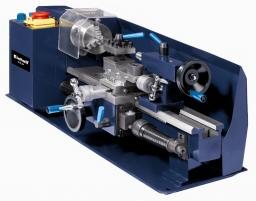# Lathe

Calculate the percentage of waste if the cube with 53 cm long edge is lathed to cylinder with a maximum volume.

Correct result:

p =  21.5 %

#### Solution:

$p = 100\% \cdot \dfrac{ V_1-V_2}{ V_1} = 100\% \cdot \dfrac{ 53^3-\pi \dfrac{ 53^2}{4} \cdot 53 }{ 53^3} = 100 \cdot (1- \dfrac{ \pi}{4} ) = 21.5 \%$We would be pleased if you find an error in the word problem, spelling mistakes, or inaccuracies and send it to us. Thank you!Tips to related online calculators
Check out our ratio calculator.

## Next similar math problems:

• LatheFrom the cube of edge 37 cm was lathed maximum cylinder. What percentage of the cube is left as waste after lathed?
• Two boxes-cubesTwo boxes cube with edges a=38 cm and b = 81 cm is to be replaced by one cube-shaped box (same overall volume). How long will be its edge?
• Cube into cylinderIf we dip a wooden cube into a barrel with a 40cm radius, the water will rise 10 cm. What is the size of the cube edge?
• Big cubeCalculate the surface of the cube, which is composed of 64 small cubes with an edge 1 cm long.
• Cube zoomHow many percents do we increase the volume and surface of the cube if we magnify its edge by 38 %?
• SeawaterSeawater has a density of 1025 kg/m3, ice 920 kg/m3. 8 liters of seawater froze and created a cube. Calculate the size of the cube edge.
• Tetrahedral prismThe height of a regular tetrahedral prism is three times greater than the length of the base edge. Calculate the length of the base edge, if you know that the prism volume is 2187 cm3.
• Cube edgesIf the edge length of the cube increases by 50%, how does the volume of this cube increase?
• Area of a cubeCalculate the surface area of a cube if its volume is equal to 729 cubic meters.
• Cylinder - areaThe diameter of the cylinder is one-third the length of the height of the cylinder. Calculate the surface of cylinder if its volume is 2 m3.
• Cuboids in cubeHow many cuboids with dimensions of 6 cm, 8 cm and 12 cm can fit into a cube with side 96 centimeters?
• Cube basicsHow long is the edge length of a cube with volume 15 m3?
• Inscribed sphereHow many % of the volume of the cube whose edge is 6 meters long is a volume of a sphere inscribed in that cube?
• Reduce of the volumeCalculate how many % reduce the volume of the cube is we reduced length of each edge by 10%.
• Sphere fallHow many percent fall volume of sphere if diameter fall 10×?
• Cube and waterHow many liters of water can fit into a cube with an edge length of 0.11 m?
• Equilateral cylinderEquilateral cylinder (height = base diameter; h = 2r) has a volume of V = 199 cm3 . Calculate the surface area of the cylinder.